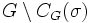# Infinite group has no non-identity finitary automorphism

## Statement

Let$G$ be an infinite group and$\sigma$ be an automorphism of$G$ that is a finitary permutation of$G$: the set of points of$G$ that are moved by$\sigma$ is finite. Then,$\sigma$ is the identity automorphism of$G$.

## Facts used

1. The set of fixed points under any automorphism$\sigma$ of$G$ is a subgroup of$G$. This subgroup is often termed the centralizer of$\sigma$, and denoted$C_G(\sigma)$.
2. Proper subgroup of infinite group is coinfinite: For an infinite group, the complement of any proper subgroup is infinite.

## Proof

Given: A group$G$, an automorphism$\sigma$ of$G$ that is a finitary permutation.

To prove:$\sigma$ is the identity automorphism.

Proof: By fact (1), the set$C_G(\sigma)$ of fixed points under$\sigma$ in$G$ is a subgroup of$G$. By the definition of finitary permutation, the complement$G \setminus C_G(\sigma)$ is a finite subset of$G$. But fact (2) tells us that if$C_G(\sigma)$ is proper, then$G \setminus C_G(\sigma)$ is infinite in size. Thus, we must have$C_G(\sigma) = G$, so$\sigma$ is the identity automorphism on$G$.